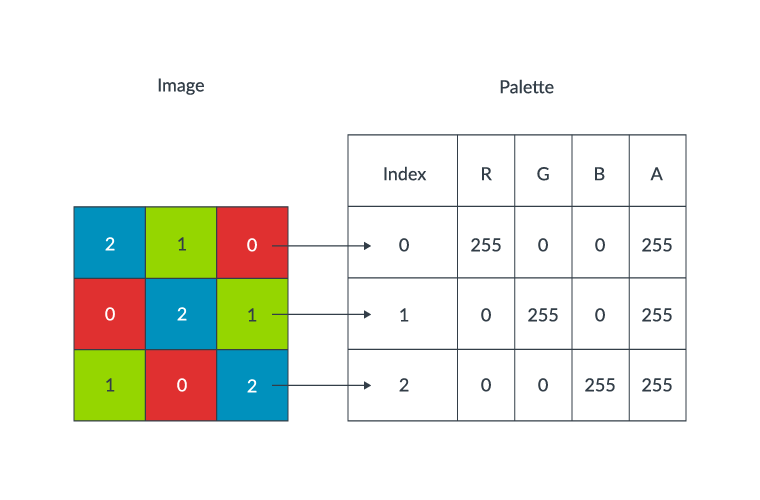## Color palette expansion

In palettized PNG images, color information is not contained directly in the image’s pixels. Instead, each pixel contains an index value into a palette of colors. This technique reduces the file size of PNG images, but means extra work must be done to display the PNG.

To render the PNG image, each palette index must be converted to an RGBA value by looking up that index in the palette. The following diagram shows how the palette maps different index values to RGB values.### Unoptimized implementation

The original implementation of the palette expansion algorithm can be found in `png_do_expand_palette()`. The code iterates over every pixel, looking up each palette index (`*sp`) and adding the corresponding RGBA values to the output stream.

```for (i = 0; i < row_width; i++)
{
if ((int)(*sp) >= num_trans)
*dp-- = 0xff;
else
*dp-- = trans_alpha[*sp];
*dp-- = palette[*sp].blue;
*dp-- = palette[*sp].green;
*dp-- = palette[*sp].red;
sp--;
}```

### Neon-optimized implementation

The optimized code uses Neon instructions to parallelize the data transfer and restructuring. The original code individually copied across each of the RGBA values from the index. The optimized code uses Neon intrinsics to construct a four-lane vector containing the R, G, B, and A values. This vector is then stored into memory. The optimized code using Neon intrinsics is as follows:

```for(i = 0; i + 3 < row_width; i += 4) {
uint32x4_t cur;
png_bytep sp = *ssp - i, dp = *ddp - (i << 2);
cur = vld1q_dup_u32 (riffled_palette + *(sp - 3));
cur = vld1q_lane_u32(riffled_palette + *(sp - 2), cur, 1);
cur = vld1q_lane_u32(riffled_palette + *(sp - 1), cur, 2);
cur = vld1q_lane_u32(riffled_palette + *(sp), cur, 3);
vst1q_u32((void *)dp, cur);
}```

Intrinsic Description
`vld1q_dup_u32` Load all lanes of a vector with the same value from memory.
`vld1q_lane_u32` Load a single lane of a vector with a value from memory.
`vst1q_u32` Store a vector into memory.

### Results

By using vectors to speed up the data transfer, performance gains in the range 10% to 30% have been observed.

This optimization started shipping in Chromium M66 and libpng version 1.6.36.

The following resources provide additional information about the `png_do_expand_palette()` optimization: